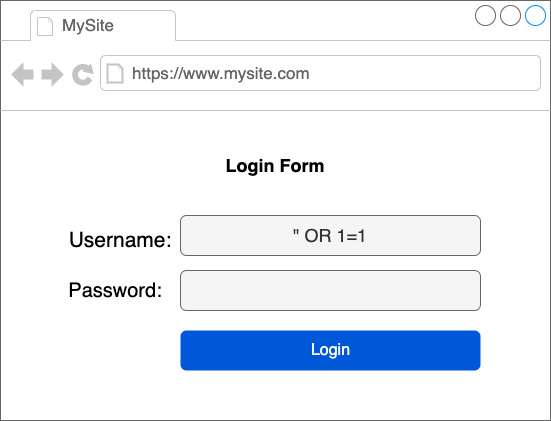# SQL Injections

## What is SQL injection?

SQL injection is a technique by which an attacker can hack your database using following methods:

• If your website has user inputs and if they are not validated properly an attacker can build harmful queries using invalid inputs.

## Examples of SQL injections

Let say that you have a website where you have login form. Assume that you do not have proper validation inplaced and you are using form fields to build database query:You take the user inputs and create sql query to get the records from database. Let's look at the following code and see how it can easily be hacked using sql injection:

```// take user inputs

// build sql query
var sqlQuery = 'select * from users where username = "' + username + '" and password = "' + password + '";

// result of above query would be
select * from users where username = "" OR 1=1 -- and password = ""```

Here both username and password are incorrect. But the query which is constructed will run and will return all user records because of condition OR 1=1.

Because -- is a comment line in SQL, everything following that will be ignored. The query will only validate between username="" OR 1=1.

Because 1=1 is always true, the user will be granted access.

You can see here that attackers they think beyond developer's mind. They get creative and find different ways to exploit the database.

## How to prevent SQL injections?

• Use of Prepared Statements (with Parameterized Queries)
• Always validate inputs from both front end and backend.
• Escaping All User Supplied Input

Prepared statements are very useful against SQL injections, because parameter values, which are transmitted later using a different protocol, need not be correctly escaped.

Example of prepared statement in PHP using MySQLi or PDO:

```// prepare and bind using MySQLi
\$stmt = \$conn->prepare("INSERT INTO users (firstname, lastname, email) VALUES (?, ?, ?)");
\$stmt->bind_param("sss", \$firstname, \$lastname, \$email);
\$stmt->execute();

// OR

// prepare sql and bind parameters using PDO library
\$stmt = \$conn->prepare("INSERT INTO users (firstname, lastname, email) VALUES (:firstname, :lastname, :email)");
\$stmt->bindParam(':firstname', \$firstname);
\$stmt->bindParam(':lastname', \$lastname);
\$stmt->bindParam(':email', \$email);
\$stmt->execute();```

# Examples of different types of SQL injections:

There are different ways sql injections are performed followings are some of the good examples:

• SQL injection based on 1=1
• SELECT * FROM users WHERE user_id = 105 OR 1=1;
• SELECT * FROM users WHERE user_name ="" OR 1=1 -- password = ""
• SQL injection based on "" = ""
• SELECT * FROM users WHERE username ="" OR "" = "" AND password ="" OR "" = ""
• SQL injection based on batch statements (;) operator
• SELECT * FROM users; DROP TABLE users;
• SELECT * FROM users WHERE id = 1; DROP TABLE users;
• SELECT * FROM users WHERE id = 1; UPDATE users SET password = 'something' where username = 'admin'--
• SQL injection based on comment -- characters
• `SELECT * FROM products WHERE category = 'soaps' OR 1=1--' AND active = 1`
• SQL injection based on union command
• SELECT * FROM products WHERE id = '1' UNION SELECT * FROM users;
• SQL injection based on hex